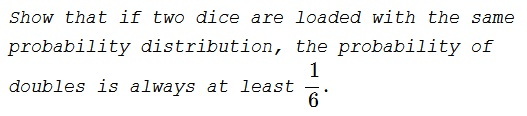# Probability of Doubles

### Problem### Solution 1

We want to show that, if $p_k,$ is the probability of getting $k$ on top, $k=1,\ldots,6$ then

$\displaystyle p_1^2+p_2^2+p_3^2+p_4^2+p_5^2+p_6^2\ge\frac{1}{6}.$

What we know is that $p_1+\ldots+p_6=1.$

\displaystyle\begin{align} 1&=(p_1+p_2+p_3+p_4+p_5+p_6)^2\\ &=(1\cdot p_1+1\cdot p_2+1\cdot p_3+1\cdot p_4+1\cdot p_5+1\cdot p_6)^2\\ &\le (1^2+1^2+1^2+1^2+1^2+1^2)(p_1^2+p_2^2+p_3^2+p_4^2+p_5^2+p_6^2) \end{align}

and the required inequality follows.

### Solution 2

Let $\{p_1, p_2,p_3,\ldots,p_6\}$ be the probability of each number on dice - for both dices. Then $p_1 + p_2 +\ldots+p_6 = 1,$ and $P (doubles) = p_1^2 + p_2^2 +\ldots+p_6^2.$ By Jensen's inequality, (since $x^2$ is convex)

\displaystyle \begin{align} \frac{P(doubles)}{6} &= \left( \frac{p_1 + p_2 + \ldots+p_6}{6} \right)^2\\ \frac{P(doubles)}{6} &\ge \left( \frac{1}{6} \right)^2\\ P(doubles) &= \frac{1}{6}. \end{align}

### Solution 3

Let $p_i$ be the probability that a die turns up $i$.

\displaystyle \begin{align} 1&=\left(\sum_i p_i\right)\left(\sum_j p_j\right)=\sum_i p_i^2 + 2 \sum_{j,k,j\neq k} p_j,p_k \\ &\leq\sum_i p_i^2 + \sum_{j,k,j\neq k} (p_j^2+p_k^2)=\sum_i p_i^2 + 5\sum_j p_j^2 =6\sum_i p_i^2. \end{align}

Thus, probability of a double is $\displaystyle \sum_i p_i^2~\geq~\frac{1}{6}.$

### Solution 4

You can as well throw one die twice. let $p_x$ be the probability of getting $x$ with one die. Then $\displaystyle \sum_x p_x^2$ is minimal when the largest $p_x^2$ is minimal as $x^2$ convex, which is when $\displaystyle p_x=\frac{1}{6}$ for all $x.$ So $\displaystyle \sum_x p_x^2 \ge \frac{1}{6}$.

### Solution 5

Let $\displaystyle \bar{e}=\left(\frac{1}{6},\frac{1}{6},\frac{1}{6},\frac{1}{6},\frac{1}{6},\frac{1}{6}\right)^T.$ Note that $\displaystyle \bar{e}^T\bar{e}=\frac{1}{6}.$ Let $\bar{x}=\bar{e}+\bar{\eta},$ where $\displaystyle \sum_i\eta_i=0.$ Then

\begin{align} \bar{x}^T\bar{x}&=\bar{e}^T\bar{e}+2\bar{e}^T\bar{\eta}+\bar{\eta}^T\bar{\eta}\\ &=\bar{e}^T\bar{e}+\bar{\eta}^T\bar{\eta}\\ &\ge \bar{e}^T\bar{e}=\frac{1}{6}. \end{align}

### Acknowledgment

This is Exercise 8.7 from Concrete Mathematics: A Foundation for Computer Science by R. L. Graham, D. E. Knuth, O. Potashnik (Addison-Wesley Publishing Company, 1994).

Solution 2 is by Kriteesh Parashar; Solution 3 is by Amit Itagi; Solution 4 is by Jacob Janssen; Solution 5 is by Ian on twitter.